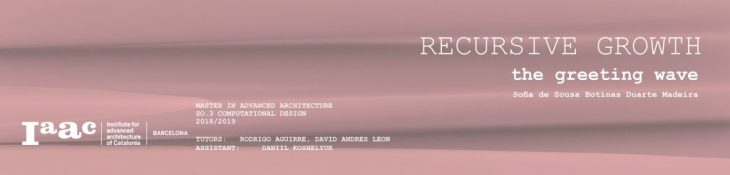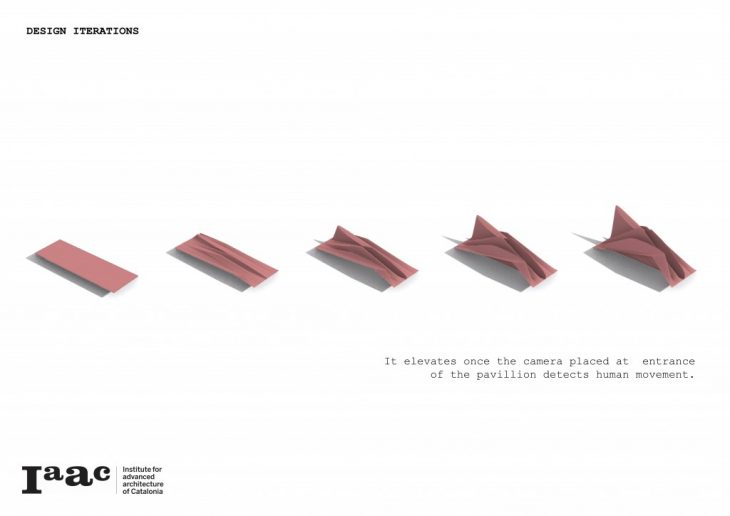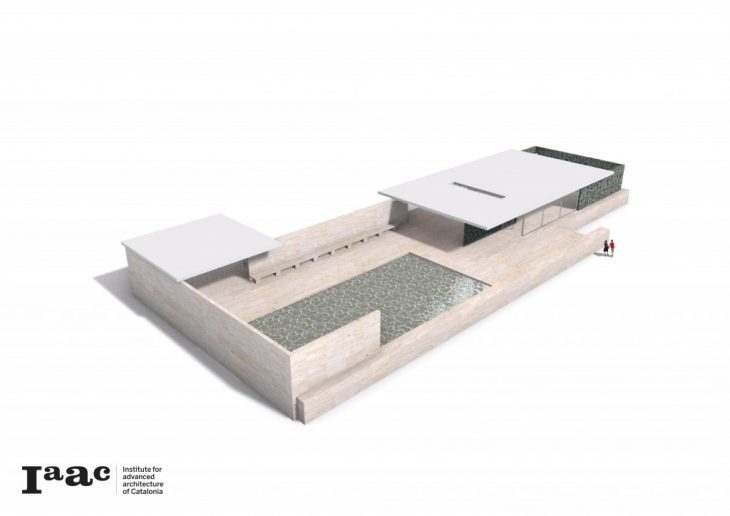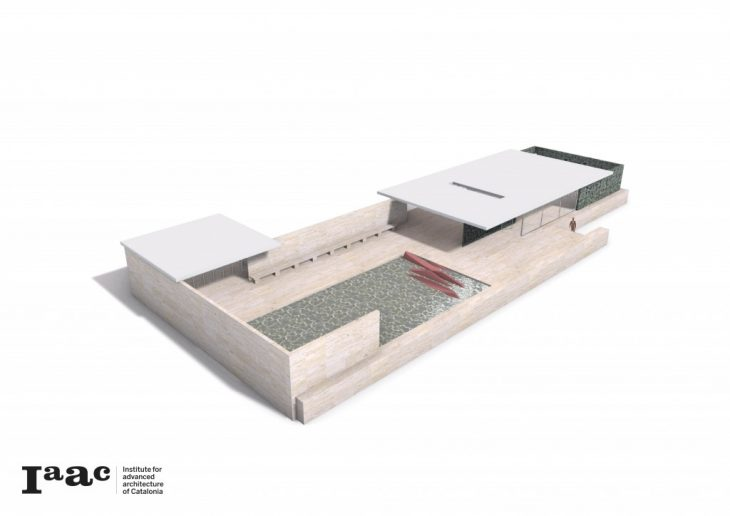As a case study students will have to intervene a part of the Mies van der Rohe pavilion and propose an architectural installation applying recursive systems which resembles how would nature proliferate in a contained environment (take over the space).
The Mies van der Rohe pavilion was designed as a building and nothing more, it would not house art or sculptures, rather the pavilion would be a place of tranquility and escape from the exposition: in effect transforming the pavilion into an inhabitable sculpture.
To contribute to this peaceful environment I decided to design a structure that would welcome the visitors upon arrival in a very smooth and mellow way. The design of my installation is a movable structure that works as a wave which raises from the reflecting pool to greet the guests and lowers down equally smoothly as they pass by, contributing to the architects desire of tranquility and serenity at the pavilion.1. Create a point at the beginning of the reflecting pool.
2. Set a point component to that same point.
3. Use the Line SDL command: the “start” will be the existing point.
4. Use a unit X component for the direction of the line and use a slider to set the length
5. Connect a move component to the line output and add a unit y direction, following a slider to define the width.
6. Use two divide curve components to create the different compartments, one for each line.
7. Use the same number slider for both number of segments.
8. Create a Line between the two output points.
9. Evaluate the curve of that line.
10. Use a range component to take each of the points from the in-between lines.
11. Use the number of segments slider previously used for the steps of this range.
12. Randomize the list with a Jitter component on which the resulting range is the list.
13. The parameter input of the evaluated curve will be the resulted values of the list.
14. To move the resulting points upwards use the move component with a unit Z direction.
15. Connect the unit Z component to a new jitter component connecting to the existing range component to follow random parameters.
16. To choose how much the points move upwards use a multiplication component with a number slider which will determine this elevation.
17. To reconnect the resulting points use a Weave component where the 1ist value will be the 1st initial line, the second will be the random points and the third the second initial line.
18. Use a 0,1,2 panel with multiline the date to create packs of 3.
19. With a polyline command connect the points to the base.
20. Connect a loft command to the polyline component and flatten the input curves.
21. Use loft options to define the type of loft.